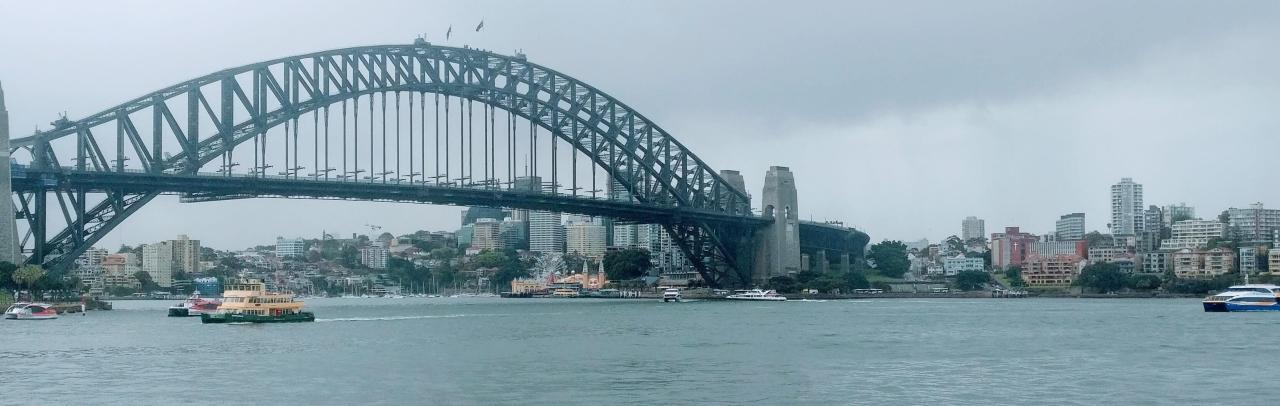# POJ1046：Color Me Less

Time Limit: 1000MS Memory Limit: 10000K
Total Submissions: 30310 Accepted: 14732

Description

A color reduction is a mapping from a set of discrete colors to a smaller one. The solution to this problem requires that you perform just such a mapping in a standard twenty-four bit RGB color space. The input consists of a target set of sixteen RGB color values, and a collection of arbitrary RGB colors to be mapped to their closest color in the target set. For our purposes, an RGB color is defined as an ordered triple (R,G,B) where each value of the triple is an integer from 0 to 255. The distance between two colors is defined as the Euclidean distance between two three-dimensional points. That is, given two colors (R1,G1,B1) and (R2,G2,B2), their distance D is given by the equation

Input

The input is a list of RGB colors, one color per line, specified as three integers from 0 to 255 delimited by a single space. The first sixteen colors form the target set of colors to which the remaining colors will be mapped. The input is terminated by a line containing three -1 values.
Output

For each color to be mapped, output the color and its nearest color from the target set.

If there are more than one color with the same smallest distance, please output the color given first in the color set.
Sample Input

0 0 0
255 255 255
0 0 1
1 1 1
128 0 0
0 128 0
128 128 0
0 0 128
126 168 9
35 86 34
133 41 193
128 0 128
0 128 128
128 128 128
255 0 0
0 1 0
0 0 0
255 255 255
253 254 255
77 79 134
81 218 0
-1 -1 -1
Sample Output

(0,0,0) maps to (0,0,0)
(255,255,255) maps to (255,255,255)
(253,254,255) maps to (255,255,255)
(77,79,134) maps to (128,128,128)
(81,218,0) maps to (126,168,9)

```#include
using namespace std;
#define n 1000
int main()
{
int r[n],g[n],b[n],flag=0;
int d=0;
int i=0,j=0,k=0;
int temp;
while (cin>>r[i]>>g[i]>>b[i],r[i]!=-1&&g[i]!=-1&&b[i]!=-1)
{
j=i;
if(j>=16)
{
d=(r[j]-r)*(r[j]-r)+(g[j]-g)*(g[j]-g)+(b[j]-b)*(b[j]-b);
for(flag=k=0;ktemp)
{
d=temp;
flag=k;
}
}
cout